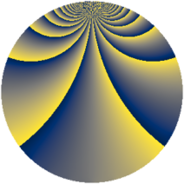# Properties

 Label 1078.2.rLevel $1078$ Weight $2$ Character orbit 1078.r Rep. character $\chi_{1078}(23,\cdot)$ Character field $\Q(\zeta_{21})$ Dimension $576$ Sturm bound $336$

# Related objects

## Defining parameters

 Level: $$N$$ $$=$$ $$1078 = 2 \cdot 7^{2} \cdot 11$$ Weight: $$k$$ $$=$$ $$2$$ Character orbit: $$[\chi]$$ $$=$$ 1078.r (of order $$21$$ and degree $$12$$) Character conductor: $$\operatorname{cond}(\chi)$$ $$=$$ $$49$$ Character field: $$\Q(\zeta_{21})$$ Sturm bound: $$336$$

## Dimensions

The following table gives the dimensions of various subspaces of $$M_{2}(1078, [\chi])$$.

Total New Old
Modular forms 2064 576 1488
Cusp forms 1968 576 1392
Eisenstein series 96 0 96

## Trace form

 $$576 q + 48 q^{4} - 4 q^{5} + 20 q^{6} + 8 q^{7} + 84 q^{9} + O(q^{10})$$ $$576 q + 48 q^{4} - 4 q^{5} + 20 q^{6} + 8 q^{7} + 84 q^{9} - 8 q^{13} + 8 q^{14} - 24 q^{15} + 48 q^{16} + 12 q^{17} + 8 q^{20} - 16 q^{21} + 4 q^{24} + 56 q^{25} - 12 q^{26} + 24 q^{27} - 4 q^{28} + 4 q^{29} + 16 q^{30} - 4 q^{31} - 4 q^{33} + 8 q^{34} - 4 q^{35} - 84 q^{36} - 8 q^{37} - 4 q^{38} - 52 q^{39} - 88 q^{41} + 48 q^{42} + 8 q^{43} + 4 q^{45} - 156 q^{46} + 44 q^{47} - 16 q^{49} - 16 q^{50} + 12 q^{51} + 32 q^{52} - 52 q^{53} - 152 q^{54} - 4 q^{56} + 92 q^{57} - 52 q^{58} - 116 q^{59} + 12 q^{60} + 36 q^{61} - 24 q^{62} + 104 q^{63} - 96 q^{64} + 28 q^{65} - 8 q^{66} + 12 q^{67} + 12 q^{68} - 24 q^{69} - 44 q^{70} + 44 q^{71} - 16 q^{73} + 12 q^{74} + 108 q^{75} - 4 q^{77} + 80 q^{78} - 4 q^{79} + 24 q^{80} + 108 q^{81} - 8 q^{82} + 44 q^{83} + 80 q^{84} + 16 q^{85} + 12 q^{86} + 12 q^{87} + 12 q^{90} - 156 q^{91} - 104 q^{93} - 8 q^{94} + 28 q^{95} + 4 q^{96} - 16 q^{97} + 48 q^{98} + O(q^{100})$$

## Decomposition of $$S_{2}^{\mathrm{new}}(1078, [\chi])$$ into newform subspaces

The newforms in this space have not yet been added to the LMFDB.

## Decomposition of $$S_{2}^{\mathrm{old}}(1078, [\chi])$$ into lower level spaces

$$S_{2}^{\mathrm{old}}(1078, [\chi]) \cong$$ $$S_{2}^{\mathrm{new}}(49, [\chi])$$$$^{\oplus 4}$$$$\oplus$$$$S_{2}^{\mathrm{new}}(98, [\chi])$$$$^{\oplus 2}$$$$\oplus$$$$S_{2}^{\mathrm{new}}(539, [\chi])$$$$^{\oplus 2}$$